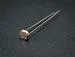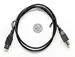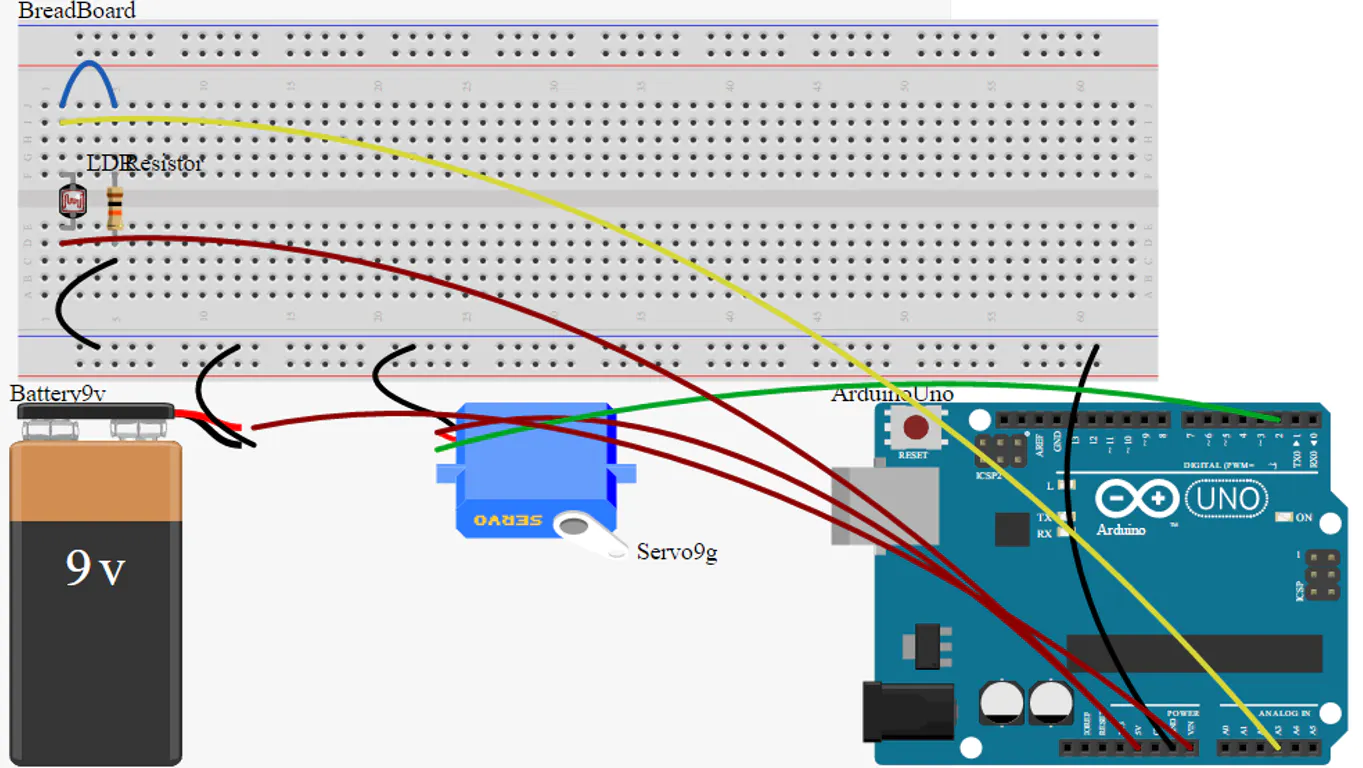# The Troll Switch

Turn Off the light, and it will turn ON: endless fun!

BeginnerFull instructions provided1 hour2,243

## Things used in this project

### Hardware componentsArduino UNO
×1×1Jumper wires (generic)
×19V battery (generic)
×19V Battery Clip
×1
 Servos (Tower Pro MG996R)
×1Photo resistor
×1Resistor 10k ohm
×1USB-A to B Cable
×1

### Software apps and online servicescircuito.ioArduino IDE

## Schematics

### The Troll Switch Circuit Diagram

The same as above, made with circuito.io## Code

### The Troll Switch

Arduino
Here's the program for the joke. Upload it to the mounted Arduino and ready to go!
```#include <Servo.h> // We need this library to operate the Servo

int LDR = A3; // We connect the LDR to the A3 pin
int light = 0; // A variable to avoid infinite moving loop
long randomTime; // We will store the time here later

Servo myservo; // We will call our servo "myservo"

void setup() {

pinMode(LDR, INPUT); // The LDR will act as an INPUT

myservo.attach(2); // We will connect the Servo to digital pin 2

Serial.begin(9600); // To start the Serial communication, useful for debugging

myservo.write(20); // At first, we need the servo at this position

}

void loop() {

if (analogRead(LDR) < 100 && light == 0) { // If the light value is less tahn 100 (and the light variable is set to 0)

randomTime = (random (0, 5) * 1000); // The time will be a random number between 0 and 5, multiplied by 1000 to make it into seconds
Serial.println(randomTime); // This will print the time (for debugging)

delay(randomTime); // The Arduino will wait that random time

myservo.write(80); // And then move the Servo to de 80 position
delay(200); // Wait for 0,2 seconds

myservo.write(20); // And move the Servo to the initial position (20)

light = 1; // This will set the "light" variable to 1
}

if (analogRead(LDR) > 99) { // If the light value is higher that 99 (A.K.A the light is on)...

light = 0; // The "light" variable will be set to 0

}

Serial.println(analogRead(LDR)); // The light value will be printed
delay(500); // Wait for half a second
}
```

## Credits

### Aitor Gamarra

2 projects • 2 followers